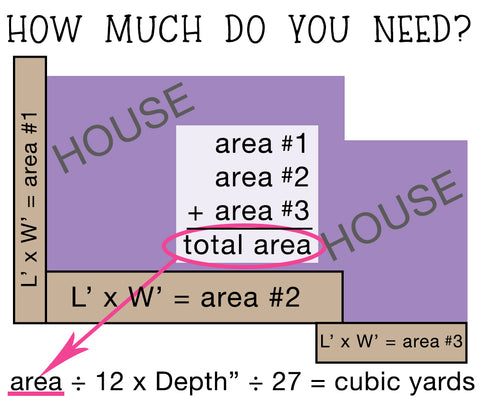# How to Calculate Cubic Yards or Tons

To convert to tons, please see the description in the sand or gravel that you are interested in for the weight per cubic yard.
Calculator placeholder

_______________________________________
use calulator below if you know your total square feet
Calculator placeholder

_______________________________________

The formula for figuring out how many cubic yards you need to cover your area isn’t difficult.Length (in feet) x Width (in feet) ÷ 12 x Depth (in inches) ÷ 27 = Cubic Yards

To figure up multiple areas, find the square footage of each area, add those together and continue with the rest of the equation:

12’ x 8’ = 96 sq ft

4’ x 7’ = 28 sq ft

9’ x 10’ = 90 sq ft

5’ x 20’ = 100 sq ft

These areas have a combined square footage of 314; so now we start the rest of the formula with dividing by 12:

314 ÷ 12 x 2” (depth in inches) ÷ 27 = 1.93 cubic yards

So for these areas, at a depth of 2”, I’d need 2 cubic yards.

This formula works the same for any material (mulch, topsoil, gravel, sand).

Note: If you have multiple areas, you cannot add all the lengths and all the widths together. That will give you wonky numbers.